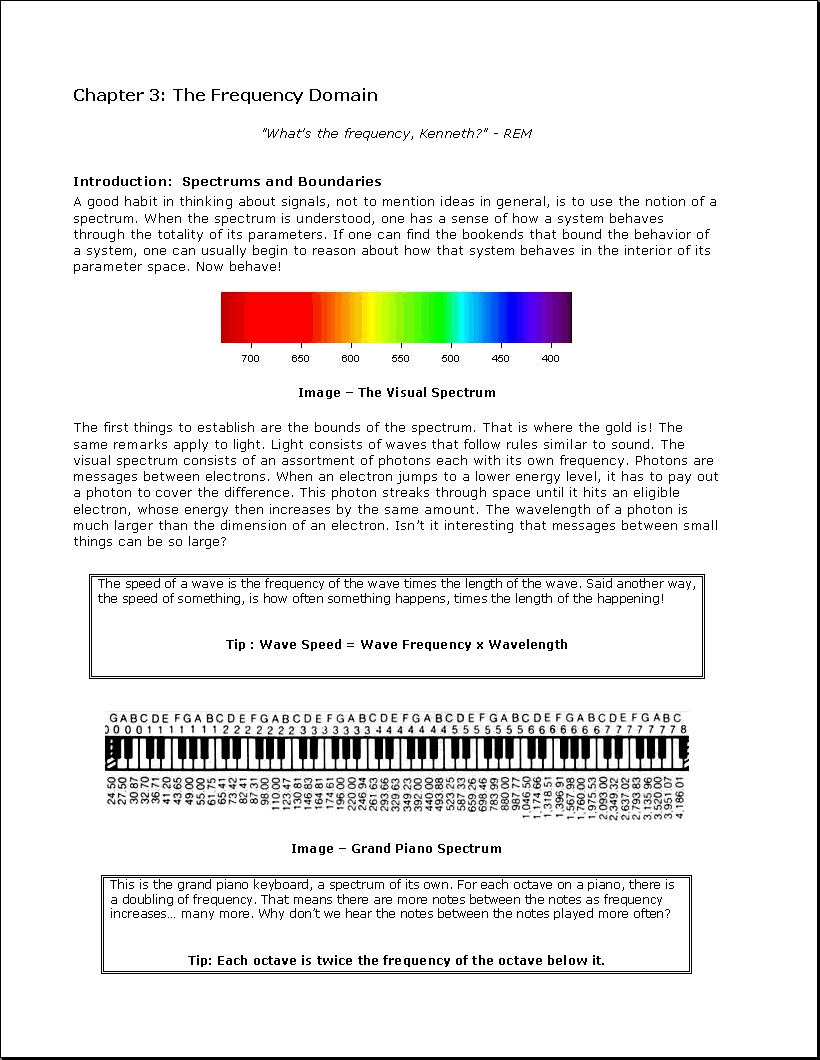Searchable plaintext: Chapter 3: The Frequency Domain " What's the frequency, Kenneth?" - REM Introduction: Spectrums and Boundaries A good habit in thinking about signals, not to mention ideas in general, is to use the notion of a spectrum. When the spectrum is understood, one has a sense of how a system behaves through the totality of its parameters. If one can find the bookends that bound the behavior of a system, one can usually begin to reason about how that system behaves in the interior of its parameter space. Now behave! Image - The Visual Spectrum The first things to establish are the bounds of the spectrum. That is where the gold is! The same remarks apply to light. Light consists of waves that follow rules similar to sound. The visual spectrum consists of an assortment of photons each with its own frequency. Photons are messages between electrons. When an electron jumps to a lower energy level, it has to pay out a photon to cover the difference. This photon streaks through space until it hits an eligible electron, whose energy then increases by the same amount. The wavelength of a photon is much larger than the dimension of an electron. Isn't it interesting that messages between small things can be so large? The speed of a wave is the frequency of the wave times the length of the wave. Said another way, the speed of something, is how often something happens, times the length of the happening! Tip : Wave Speed = Wave Frequency x Wavelength Image - Grand Piano Spectrum This is the grand piano keyboard, a spectrum of its own. For each octave on a piano, there is a doubling of frequency. That means there are more notes between the notes as frequency increases... many more. Why don't we hear the notes between the notes played more often? Tip: Each octave is twice the frequency of the octave below it.